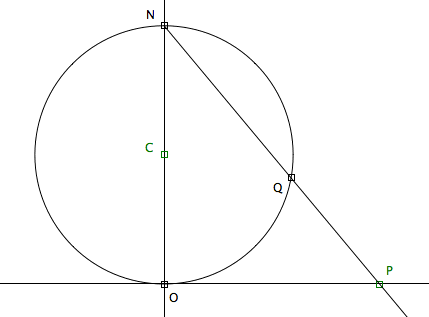# Sphere Projection

By Xah Lee. Date:Cross section of a Sphere Projection. _z_sphere_proj.html

Let there be a sphere of radius 1 sitting on top of the plane. Now, let there be a point P on the plane. Let there be a line L passing the sphere's north pole N to the point P. This line will intersect the sphere at a point Q. In this way, every point P on the plane will have a corresponding point Q on the sphere. We call this process the Sphere Projection.

(note: this is traditionally called Stereographic projection, and the study of complex numbers by this project on the sphere is generalled called Riemann Sphere.)

## The Formula for Coordinate Correspondence

Let the coordinate of P be {p1,p2}. Let the coordinate of Q be {q1,q2,q3}. We want to find the formula of Q expressed in terms of p1, p2.

The equation of a sphere sitting on origin with radius r is

```x^2+y^2+(z-r)^2-r^2==0
```

The parametric formula for a line in the direction of P-N and passing N is:

```({p1,p2,p3}-{n1,n2,n3})t+{n1,n2,n3}
```
```Rewrite this as:
x==n1+(-n1+p1) t
y==n2+(-n2+p2) t
z==n3+(-n3+p3) t
```

replace x,y,z in the sphere equation by these, and we get a quadratic equation in t. Solving for t, then plug the solution of t back to the parametric equation of a line, will get us the formula for the coordinates of points that is the intersection of the line and the sphere. The symbolic formula is several lines long ( line_sphere_sol.txt ). In our case, p3 == 0, n1==n2==0, n3=2 r, r==1/2, then we have

{{0, 0, 1}, {p1/(1 + p1^2 + p2^2), p2/(1 + p1^2 + p2^2), 1 - 1/(1 + p1^2 + p2^2)}}

The first point is just the north pole. The second point is the formula we sought.

## Sphere Projection and 3D-Inversion

A Sphere Projection is a instance of 3D-inversion.

Imagine a Sphere with radius 2, centered on N.

## Angles are Unchanged

Theorem: Angles are preserved under Sphere Projection.

Explanation: Two different lines makes 4 angles on the plane. Suppose they are a,b,c,d. Let P denote the point they cross. Now, after sphere projection, these two lines become two curves on the sphere. They still cross at point P. The angle formed by two curves at their crossing point P is defined as the angle the two tangents at the crossing point. The theorem says, the angles are still a,b,c,d.

Proof: We know that lines are mapped into circles. If the two lines are not parallel, their image on the sphere will be two circles that cross each other. If two circles on a sphere cross each other, they will from a pair of symmetric intersections. We know these two circles pass thru north pole N, so N is one of their intersection. Now, the angles made by two circes are the same for the two intersections, so we can just look at the intersection at the N. Suppose the two lines are l1 and l2, and their images are c1 and c2. The tangent of c1 at N will be parallel to l1, similarly for c2 and l2. Therefore, the angles are preserved.

## Effects of Sphere's Position

(some author uses a sphere centered on origin, instead of sitting on origin. Here we compare their effects.)

## Simple Transformations on the Sphere and Invariant Curves

Loxodromic

### Translation

2006-11

If you have a question, put \$5 at patreon and message me.